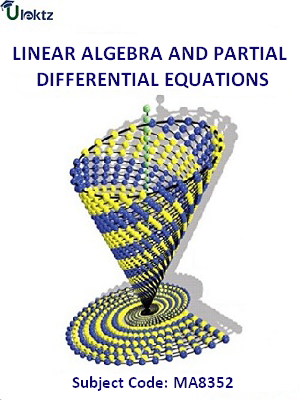uLektz apps

•My WalletMy Order
•My Profile
•My Connections
•My Books
•My Videos
•My Tests
•My Calender
•My Messages
•My Shopping Cart
•My Orders
•Account Settings
•Help

uLektz apps

# Book Details# Linear Algebra and Partial Differential Equations

Course Code:MA8352

Author:uLektz

University:

Regulation:2017

Categories:Electronics & Communication

Format :ePUB3 (DRM Protected)

Type :eBook

FREE

Description :Linear Algebra and Partial Differential Equations of MA8352 covers the latest syllabus prescribed by Anna University, Tamil Nadu for regulation 2017. Author: uLektz, Published by uLektz Learning Solutions Private Limited.

Note : No printed book. Only ebook. Access eBook using uLektz apps for Android, iOS and Windows Desktop PC.

##### Topics
###### UNIT I VECTOR SPACES

1.1 Vector spaces - Subspaces

1.2 Linear combinations and linear system of equations

1.3 Linear independence and linear dependence

1.4 Bases and dimensions

###### UNIT II LINEAR TRANSFORMATION AND DIAGONALIZATION

2.1 Linear transformation

2.2 Null spaces and ranges

2.3 Dimension theorem

2.4 Matrix Representation of a Linear Transformations

2.5 Eigenvalues and eigenvectors

2.6 Diagonalizability

###### UNIT III INNER PRODUCT SPACES

3.1 Inner product norms

3.2 Gram-Schmidt orthogonalisation process

3.3 Adjoint Operator - Least square approximation

###### UNIT IV PARTIAL DIFFERENTIAL EQUATIONS

4.1 Formation

4.2 Solutions of first order equations

4.3 Standard types and equations reducible to standard types, Equations reducible to standard forms

4.4 Singular solutions – Lagrange linear equation – Integral surface passing through a given curve

4.5 Classification of PDEs -Solution of linear equations of higher order with constant coefficients – Linear non-homogeneous partial differential equations.

###### UNIT V FOURIER SERIES SOLUTIONS OF PARTIAL DIFFERENTIAL EQUATIONS

5.1 Dirichlet conditions - General Fourier series

5.2 Half range sine and cosine series:

5.3 Method of separation of variables - Solutions of one dimensional wave equation and one-dimensional heat equation

5.4 Steady state solution of two-dimensional heat equation - Fourier series solutions in Cartesian coordinates.

### Related Books